Courses

# Moment of Inertia Tensor - Collisions, Classical Mechanics, CSIR-NET Physical Sciences Physics Notes | EduRev

## Physics for IIT JAM, UGC - NET, CSIR NET

Created by: Akhilesh Thakur

## Physics : Moment of Inertia Tensor - Collisions, Classical Mechanics, CSIR-NET Physical Sciences Physics Notes | EduRev

The document Moment of Inertia Tensor - Collisions, Classical Mechanics, CSIR-NET Physical Sciences Physics Notes | EduRev is a part of the Physics Course Physics for IIT JAM, UGC - NET, CSIR NET.
All you need of Physics at this link: Physics

## The inertia tensor

Last time, we started looking at the motion of collections of particles, and found that for a number of very interesting quantities, there is a clean division between the motion of the center of mass (CM), and the motion relative to the CM. In particular, we saw that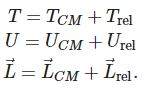We specialized further to rigid bodies, which are collections in which none of the particles move relative to each other; this allows us to treat Urel as an ignorable constant, and leaves rotation as the only possible relative motion contributing to  T and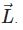After that, we studied motion about a fixed axis, say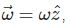and derived the familiar formulas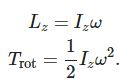Here Iz is the familiar moment of inertia for rotation about this axis. The moment of inertia tells us how resistant the object is to changes in rotational velocity; the larger Iz, the more energy we need to put in to increase ω  and Lz. (The moment of inertia plays the same role in rotation that the ordinary mass mm does in linear motion.)

But we found that in general, these are simplified relations that only hold for highly symmetric objects! As we saw, it’s often the case that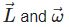don’t even point in the same direction. This means that the moment of inertia must in fact be an operator which can rotate one vector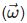into another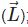in other words, it’s a matrix,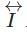Now let’s move on to rotation about an arbitrary axis, and see the general form of this inertia matrix. Take a general rotation vector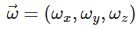the rotation is taken with respect to the center of mass and the body is otherwise stationary, so the speed of each particle is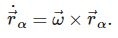Thus, the angular momentum carried by each particle is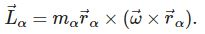Expanding out both cross products in turn is tedious, so I’ll just skip to the result, which can be written in matrix form: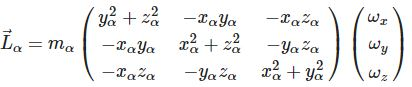This is a compact way of writing three equations, one for each component of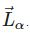Notice that setting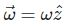gives us the fixed-axis result we found last time. Once again, to get the total angular momentum, we sum over the individual particles α, and we can pull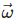out of the sum, so finally we have: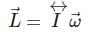which defines the inertia matrix or inertia tensor,

Definition: the inertia tensor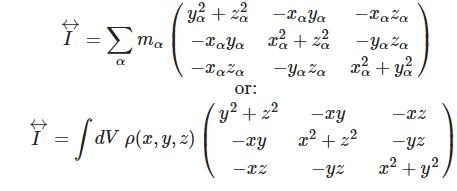The second version of this just replaces the sum with an integral, for an object described by some continuous density function ρ(x,y,z). Don’t be confused by the “integral of a matrix”; the components of the matrix are all separate, so you can think of writing nine separate integrals inside the matrix, I just wrote it like this to save space.

Notice that this is a symmetric matrix; that has some important implications, but one thing we can see immediately is that although it’s a 3x3 matrix, there are only six independent entries we have to find.

It’s very important to remember that this formula defines the moments of inertia only for rotational axes which pass through the origin of the coordinate system, x=y=z=0. This special point through which all rotational axes pass is called the pivot point. If we want to calculate rotation around an axis which doesn’t pass through the origin, we have to shift our coordinates to use the formula above. Remember, angular momentum is sensitive to where we put our coordinates! (The actual motion of the system that we find in the end will, of course, be independent of coordinate choices, but as we saw last time, statements like "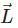is constant” do depend on coordinates.)

By the way, it shouldn’t surprise you that the equation for kinetic energy is modified too! The derivation is a bit of a pain, so I’ll just tell you that the general formula is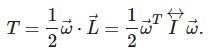You can see that this reproduces the simplified formula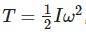which is valid only for rotation that keeps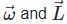parallel.

### Tensors and index notation

It’s worth making a brief detour here into index notation, which will give us a much nicer-looking formula for the inertia tensor. We know that we can break any vector down into its components: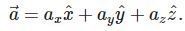Here we’ve written the components as ai, where i is a placeholder for any of x,y,z. (By convention, latin indices i,j,k...  are used for spatial coordinates.) Index notation gives us a compact way of rewriting certain formulas in terms of components. For example, the dot product: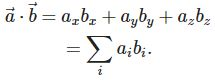This notation is easily extended to larger objects. The inertia tensor itself is a matrix, so we need two indices ij to write out its 9 components. Which entry is which, in this notation? Well, we can write out matrix-vector products as sums over indices. These two equations have the same meaning: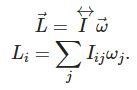So, for example, the xx-component of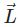is given by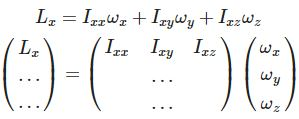This is the dot product of the column vector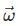with the first row of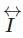, so we see that the first index labels the row, and the second index is the column.

With this notation set up, we can write a much more compact formula for the inertia tensor:

#### Inertia tensor (compact form)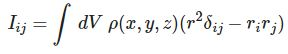where ri=(rx,ry,rz)=(x,y,z) , and r2=x2+y2+z2 (this is all still in rectangular coordinates!) I have introduced a special symbol here known as the Kronecker delta symbol, which is equal to 1 if the two indices are equal and 0 otherwise: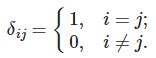In matrix form, the Kronecker delta is just the identity matrix. We could go much further with index notation; for example, I could define the cross product using a different special tensor. But for now, we only really wanted the compact version of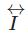so let’s move on.

By the way, I haven’t really defined the term tensor. A tensor is a mathematical object that is sensitive to coordinate changes in a certain way. Consider the simple change of coordinates from rectangular to cylindrical:

x = r cos θ

y = r sin θ

z = z

If we’re just interested in a quantity like the kinetic energy, then we just substitute in one coordinate for another using these equations: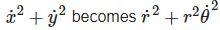for example. But if we have a vector and we want to change coordinates; we have to go further, and account for the change of basis vectors from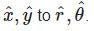A vector is an example of a tensor, because of this extra sensitivity to the unit vectors in our coordinate change. The “inertia tensor” will also depend on the unit vectors, but in a more complicated way: to fully change coordinates here, we have to identify what Irr is in terms of the original x and y components, and so on. We’re not going to deal with coordinate changes of tensors in full generality here; just keep in mind that the inertia matrix isn’t just a fixed set of numbers, but that it depends intricately on the definition of our coordinates. I’ll continue to say “inertia tensor” since that’s standard jargon, but you can just think “inertia matrix”.

### Example: inertia tensor of a cube

That’s it for index notation, for now. Let’s do an example! Cube of density ρ, side length b, rotating about one of its corners. What is the inertia tensor?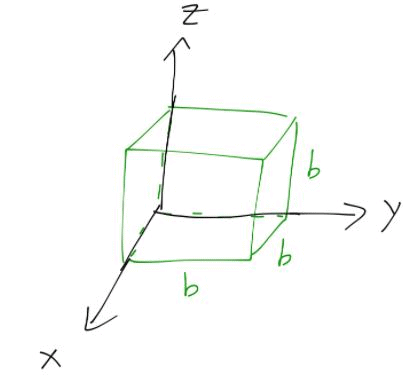Before we begin, let’s think about whether we really need to evaluate all six integrals in this case.

Let’s start with the top left diagonal component. Since ρ is constant, we can pull it out of integral: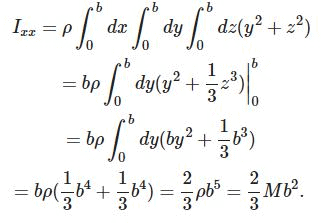where at the last step I’ve identified that the density of the cube can be rewritten as M=ρb3. Now, the off-diagonal component: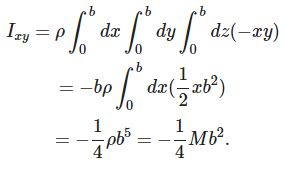All off-diagonal components are the same, and all diagonal components too, thanks to the symmetry of the cube! So the full tensor is: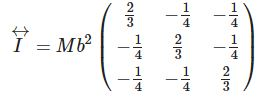As we’ve just seen, it’s very helpful to identify the symmetries of our object before we start calculating integrals! Often you’ll be able to demonstrate using symmetry either that some components of the inertia tensor are equal to each other, or even that they must be zero (for the off-diagonal components.)

One other important aside to note: moments of inertia for a compound object add together. This is easy to see from the definition: suppose we have a compound object that we can write as the sum of two densities, ρ=ρa+ρb. Then the total moment of inertia is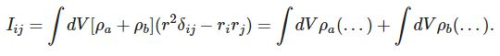So if you can split something into a small number of simple shapes, you can still easily compute its inertia tensor. The canonical example is the planet Saturn: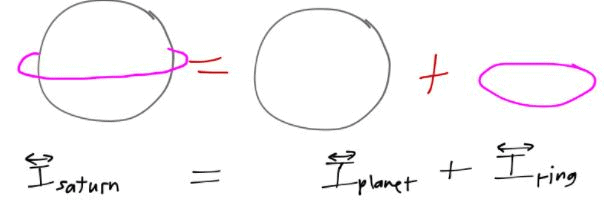This trick works just as well with negative densities, too. For example, a hollow sphere can be treated by finding the inertia tensor for a large sphere, and subtracting the inertia tensor of a smaller sphere from it.

Once again, I’ll stress that the location of the pivot point is important! You know from intro physics that a cylinder has a different moment of inertia for rotation about its edge or about its center, for example. In our cube example above, we can only use the expression we found for rotations that pass through the corner of the cube; for other pivot points,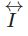will change!

Let’s see explicitly what the difference is, by recomputing the cube’s inertia tensor from its center of mass.

#### Example: inertia tensor of a cube about the CM

Much like the cube about its corner, the high degree of symmetry here means we only need to do two out of nine possible integrals. Let’s look at a diagonal element first: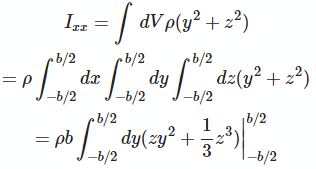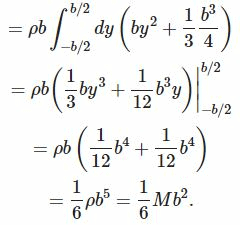On the other hand, all the off-diagonal moments are zero, for example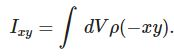This is an odd function of x and y, and our integration is now symmetric about the origin in all directions, so it vanishes identically. So the inertia tensor of the cube about its center is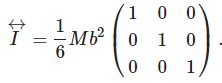### Torque-free rotational motion

We’ve seen cases so far where the inertia tensor is diagonal, and some where it isn’t. For a diagonal inertia tensor, like the cube spinning about its CM, the angular momentum is easy to find; for rotation about any of the three axes, the angular momentum vector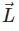points in the same direction as the angular velocity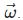. In other words,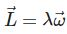where the factor λ is just the moment of inertia of the object about that axis. What does this mean in terms of the motion?

Recall that there is an equivalent to Newton’s third law for rotational motion. In intro physics, you’ve probably seen it written as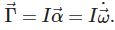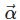is the angular acceleration; it’s just the derivative of the angular velocity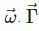is the torque, which is given by an off-center force: mathematically,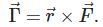For a rigid body,is the vector between the pivot point or axis of rotation and the applied force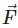. Since it’s a cross product, ifpoints towards the pivot point, or axis of rotation, or center of mass for a free body, then there is no torque, and the only resulting motion is linear.

Obviously, we need to update the freshman physics equation for motion; the expression I wrote above is just for rotation about a fixed axis with a single moment of inertia, but we know that in general the moment of inertia is a 3x3 tensor. For linear motion, Newton’s second law takes the more general form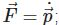this tells us manifestly that with no external forces, linear momentum is conserved. Maybe we should write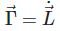in exactly the same way?.

159 docs

,

,

,

,

,

,

,

,

,

,

,

,

,

,

,

,

,

,

,

,

,

,

,

,

,

,

,

;